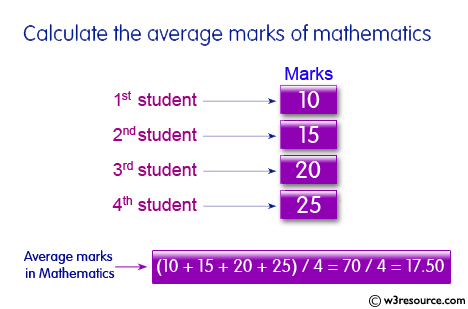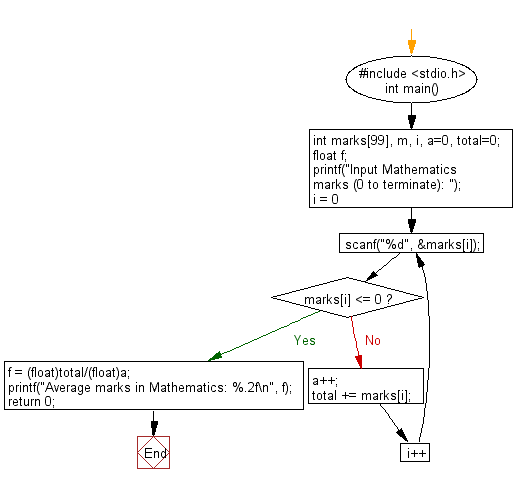﻿ C : Calculate the average mathematics marks of some students

# C Exercises: Calculate the average marks of mathematics of some students

## C Basic Declarations and Expressions: Exercise-44 with Solution

Write a C program to calculate the average mathematics marks of some students. Input 0 (excluding to calculate the average) or a negative value to terminate the input process.

Pictorial Presentation:Sample Solution:

C Code:

``````#include <stdio.h>
int main() {
int marks, m, i, a=0, total=0;
float f;

// Prompt for user input
printf("Input Mathematics marks (0 to terminate): ");

// Loop to get input marks
for(i = 0; ; i++) {
scanf("%d", &marks[i]);
if(marks[i] <= 0) {
break;
}
a++;
total += marks[i];
}

// Calculate and print average
f = (float)total/(float)a;
printf("Average marks in Mathematics: %.2f\n", f);

return 0;
}
``````

Sample Output:

```Input Mathematics marks (0 to terminate): 10
15
20
25
0
Average marks in Mathematics: 17.50
```

Flowchart:C programming Code Editor:

What is the difficulty level of this exercise?

Test your Programming skills with w3resource's quiz.

﻿